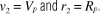Math Matters - Spacecraft - Maplesoft

# SpacecraftAlthough the science and mathematics behind space exploration is mind-boggling, many key concepts relate back to the fundamentals that many of us are familiar with. For example, modern orbital mechanics calculations still rely directly on the work of Kepler and Newton, among others.

### Sample vehicle launch calculation:

For a particular orbit segment fromto, the geometry of elliptical orbits (as proposed by Kepler) yields,Using Newton’s law of universal gravitation (for satellite mass m and the Earth’s mass M) and law of conservation of energy,Some key calculations are performed when the distance
between the two masses is at a minimum (periapsis) or a
maximum (apoapsis). For periapsis,.
Rearranging the above equations gives,Solving forgives altitude predictions.Johannes Kepler
(German, 1546-1601)
derived mathematical models (ellipses) for orbital motions.Isaac Newton
(English, 1643-1727)
developed the laws of motion and gravity. He also developed integral calculus and differential calculus, which are probably the most important fields of mathematics in modern science.Gottfried Leibniz
(German, 1646-1716)
independently developed integral and differential calculus. His notation and terminology is the standard today.